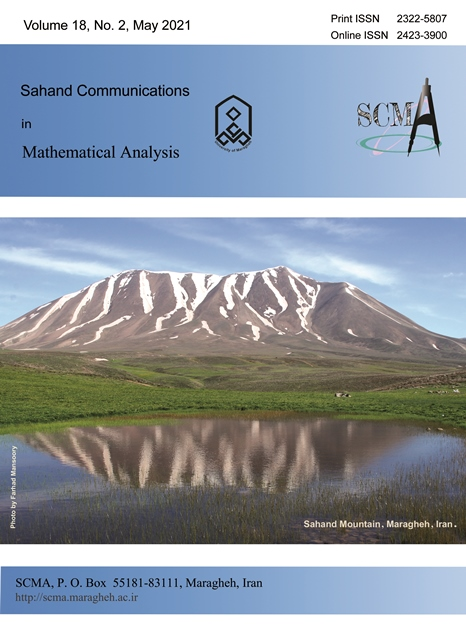Publisher: U of Maragheh(Total: 1 journals)   [Sort by number of followers]

 Showing 1 - 1 of 1 Journals sorted alphabetically Sahand Communications in Mathematical AnalysisSimilar JournalsSahand Communications in Mathematical AnalysisNumber of Followers: 0Open Access journal ISSN (Print) 2322-5807 - ISSN (Online) 2423-3900 Published by U of Maragheh[1 journal]
• Coincidence Point Results for Different Types of $H_b^{+}$-contractions
on $m_b$-Metric Spaces

• Abstract: In this paper, we give some properties of $m_b$-metric topology and prove Cantor's intersection theorem in $m_b$-metric spaces. Moreover, we introduce some newclasses of $H_b^+$-contractions for a pair of multi-valued and single-valued mappings and discuss their coincidence points. Some examples are provided to justify the validity of our main results.

• Joint Continuity of Bi-multiplicative Functionals

• Abstract: For Banach algebras $\mathcal{A}$ and $\mathcal{B}$, we show that if $\mathfrak{A}=\mathcal{A}\times \mathcal{B}$ is unital, then each bi-multiplicative mapping from $\mathfrak{A}$ into a semisimple commutative Banach algebra $\mathcal{D}$ is jointly continuous. This conclusion generalizes&nbsp; a famous result due to$\check{\text{S}}$ilov, concerning the automatic continuity of homomorphisms between Banach algebras. We also prove that every $n$-bi-multiplicative functionals on $\mathfrak{A}$ is continuous if and only if it is continuous for the case $n=2$.&nbsp;

• Fixed Point Theorems for Geraghty Type Contraction Mappings in Complete
Partial $b_{v}\left( s\right)$-Metric Spaces

• Abstract: In this paper, necessary and sufficient conditions for the existence and uniqueness of fixed points of generalized Geraghty type contraction mappings are given in complete partial $b_{v}(s)$-metric spaces. The results are more general than several results that exist in the literature because of the considered space. A numerical example is given to support the obtained results. Also, the existence and uniqueness of the solutions of an integral equation has been verified considered as an application.

• Some Properties of Complete Boolean Algebras

• Abstract: The main result of this paper is a characterization of the strongly algebraically closed algebras in the&nbsp; lattice of all real-valued continuous functions and the equivalence classes of $\lambda$-measurable. We shall provide conditions&nbsp; which strongly algebraically closed algebras carry a strictly positive Maharam submeasure. Particularly, it is proved that if $B$ is a strongly algebraically closed lattice&nbsp; and $(B,\, \sigma)$ is a Hausdorff space&nbsp; and $B$ satisfies&nbsp; the&nbsp;&nbsp; $G_\sigma$ property, then $B$ carries a strictly positive Maharam submeasure.

• Second Module Cohomology Group of Induced Semigroup Algebras

• Abstract: For a discrete semigroup $S$ and a left multiplier operator&nbsp; $T$ on&nbsp; $S$, there is a new induced semigroup $S_{T}$, related to $S$ and $T$. In this paper, we show that if $T$ is multiplier and bijective,&nbsp; then the second module cohomology groups $\mathcal{H}_{\ell^1(E)}^{2}(\ell^1(S), \ell^{\infty}(S))$ and $\mathcal{H}_{\ell^1(E_{T})}^{2}(\ell^1({S_{T}}), \ell^{\infty}(S_{T}))$ are equal, where $E$ and&nbsp; $E_{T}$ are subsemigroups of idempotent elements in $S$ and $S_{T}$,&nbsp;&nbsp; respectively.&nbsp; Finally, we show thet, for every odd $n\in\mathbb{N}$,&nbsp; $\mathcal{H}_{\ell^1(E_{T})}^{2}(\ell^1(S_{T}),\ell^1(S_{T})^{(n)})$ is a Banach space, when $S$ is a commutative inverse semigroup.

• Two Equal Range Operators on Hilbert $C^*$-modules

• Abstract: In this paper, number of properties, involving invertibility, existence of Moore-Penrose inverse and etc for modular operators with the same ranges on Hilbert $C^*$-modules&nbsp; are presented. Natural decompositions of operators with closed range enable us to find some relations of the product of operators with&nbsp; Moore-Penrose inverses under the condition that they have&nbsp; the same ranges&nbsp; in Hilbert $C^*$-modules. The triple reverse order law and the mixed reverse order law in the special cases are also given. Moreover, the range property and Moore-Penrose inverse are illustrated.

• Using Frames in Steepest Descent-Based Iteration Method for Solving
Operator Equations

• Abstract: In this paper, by using the concept of frames, two iterative methods are constructed to solve the operator equation $Lu=f$ where $L:H\rightarrow H$ is a bounded, invertible and self-adjoint linear operator on a separable Hilbert space $H$. These schemes are analogous with steepest descent method which is applied on a preconditioned equation obtained by frames instead. We then investigate their convergence via corresponding convergence rates, which are formed by the frame bounds. We also investigate the optimal case, which leads to the exact solution of the equation. The first scheme refers to the case where $H$ is a real separable Hilbert space, but in the second scheme, we drop this assumption.

• Some Common Fixed Point Results for Generalized
$\alpha_*$-$\psi$-contractive Multi-valued Mappings on Ordered Metric
Spaces with Application to Initial Value Problem

• Abstract: In 2012, Samet, et al. introduced the notion of $\alpha$-$\psi$-contractive type mappings. They have been establish some fixed point theorems for the mappings on complete metricspaces. In this paper, we introduce the notion of generalized $\alpha_*$-$\psi$-contractive multi-valued mappings and we give some related fixed point results on ordered metric spaces via&nbsp; application to an initial value problem.

• Interior Schauder-Type Estimates for Higher-Order Elliptic Operators in
Grand-Sobolev Spaces

• Abstract: In this paper&nbsp; an elliptic operator of the $m$-th order&nbsp; $L$ with continuous coefficients in the $n$-dimensional domain $\Omega \subset R^{n}$ in the non-standard Grand-Sobolev space $W_{q)}^{m} \left(\Omega \right)\,$ generated by the norm $\left\ \, \cdot \, \right\ _{q)}$ of the Grand-Lebesgue space $L_{q)} \left(\Omega \right)\,$ is considered.&nbsp; Interior&nbsp; Schauder-type estimates&nbsp; play a very important role in solving the Dirichlet problem for the equation $Lu=f$. The considered non-standard spaces are not separable, and therefore, to use classical methods for treating solvability problems in these spaces, one needs to modify these methods. To this aim, based on the shift operator, separable subspaces of these spaces are determined, in which finite infinitely differentiable functions are dense.&nbsp; Interior&nbsp; Schauder-type estimates&nbsp; are established with respect to these subspaces. It should be noted that Lebesgue spaces $L_{q} \left(G\right)\,$ are strict&nbsp;&nbsp; parts of these subspaces. This work is a continuation of the authors&nbsp; of the work \cite{28}, which established the solvability in the small of higher order elliptic equations in grand-Sobolev spaces.